# How to Calculate and Solve for Standing Bubble Point Parameter | The Calculator Encyclopedia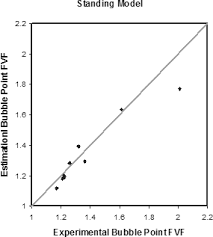The image above represents standing bubble point parameter.

To compute for the standing bubble point parameter, two essential parameters are needed and these parameters are API Gravity (°API) and Temperature (°Rankine) (T).

The formula for calculating standing bubble point parameter:

a = [0.00091(T – 460)] – [0.0125(°API)]

Where:

a = Standing Bubble Point Parameter, a
°API = API Gravity
T = Temperature (°Rankine)

Let’s solve an example;
Find the standing bubble point parameter when API Gravity is 32 and the temperature is 146.

This implies that:

°API = API Gravity = 32
T = Temperature (°Rankine) = 146

a = [0.00091(T – 460)] – [0.0125(°API)]
a = [0.00091(146 – 460)] – [0.0125(32)]
a = [0.00091(-314)] – [0.0125(32)]
a = [-0.28574] – [0.0125(32)]
a = [-0.28574] – [0.4]
a = -0.68574

Therefore, the standing bubble point parameter, a is -0.68574.

Nickzom Calculator – The Calculator Encyclopedia is capable of calculating the standing bubble point parameter.

To get the answer and workings of the standing bubble point parameter using the Nickzom Calculator – The Calculator Encyclopedia. First, you need to obtain the app.

You can get this app via any of these means:

To get access to the professional version via web, you need to register and subscribe for NGN 1,500 per annum to have utter access to all functionalities.
You can also try the demo version via https://www.nickzom.org/calculator

Apple (Paid) – https://itunes.apple.com/us/app/nickzom-calculator/id1331162702?mt=8
Once, you have obtained the calculator encyclopedia app, proceed to the Calculator Map, then click on Petroleum under EngineeringNow, Click on Fluid Properties under Petroleum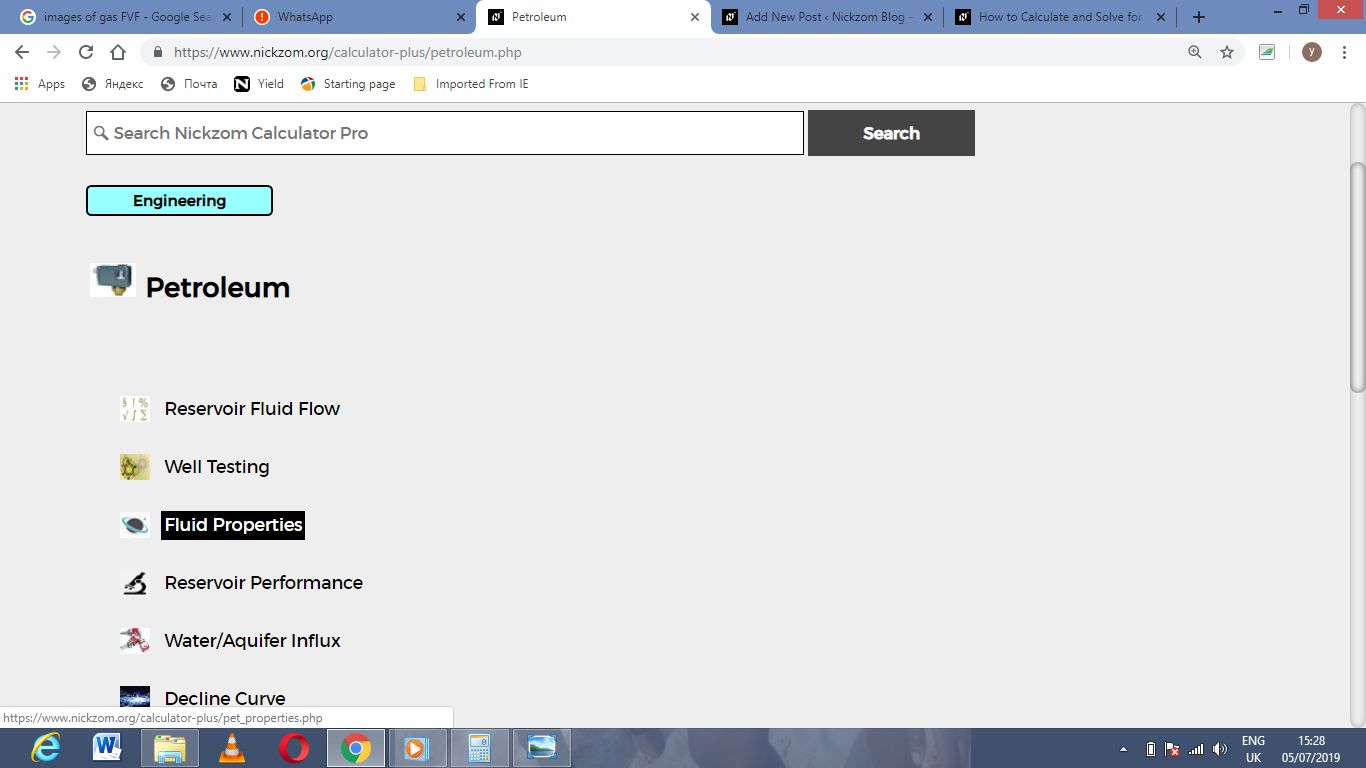Now, Click on Standing Bubble Point Parameter under Fluid Properties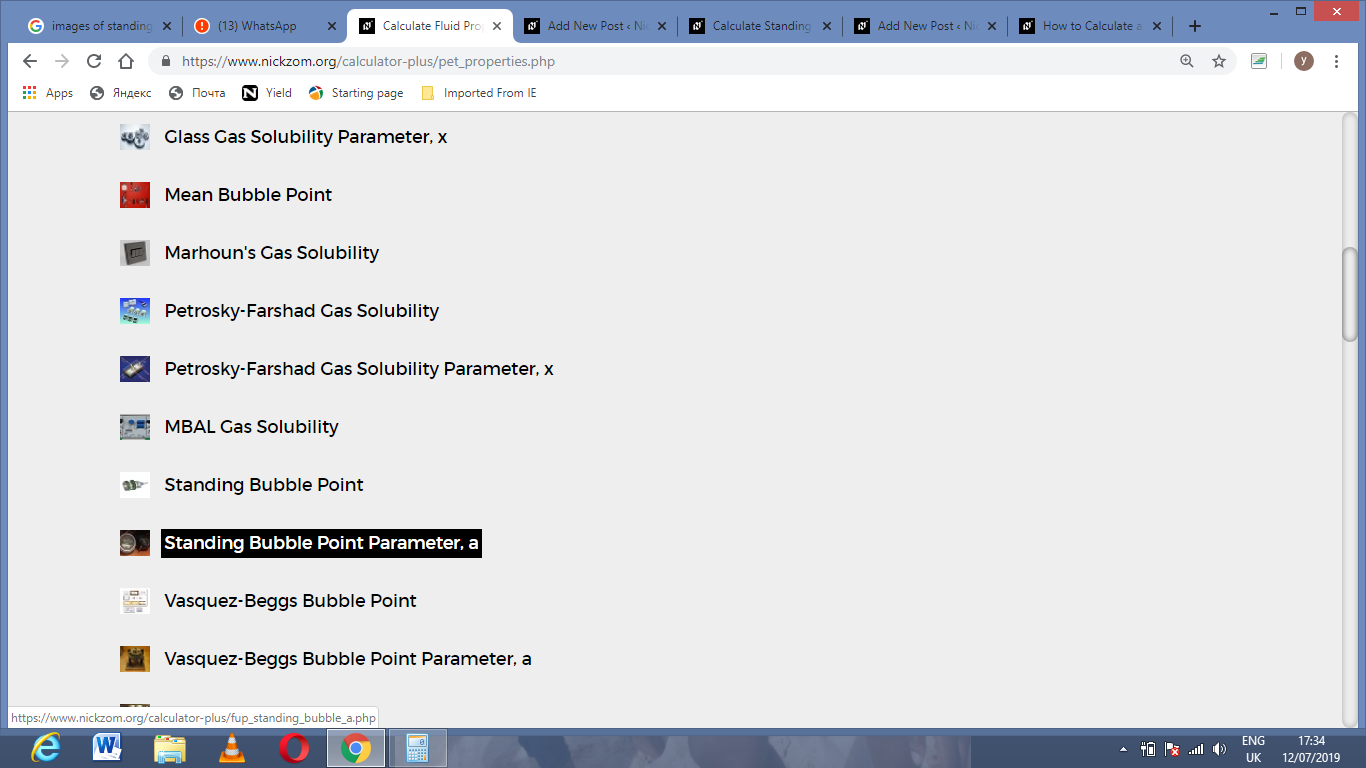The screenshot below displays the page or activity to enter your values, to get the answer for the standing bubble point parameter according to the respective parameters which are the API Gravity (°API) and Temperature (°Rankine) (T).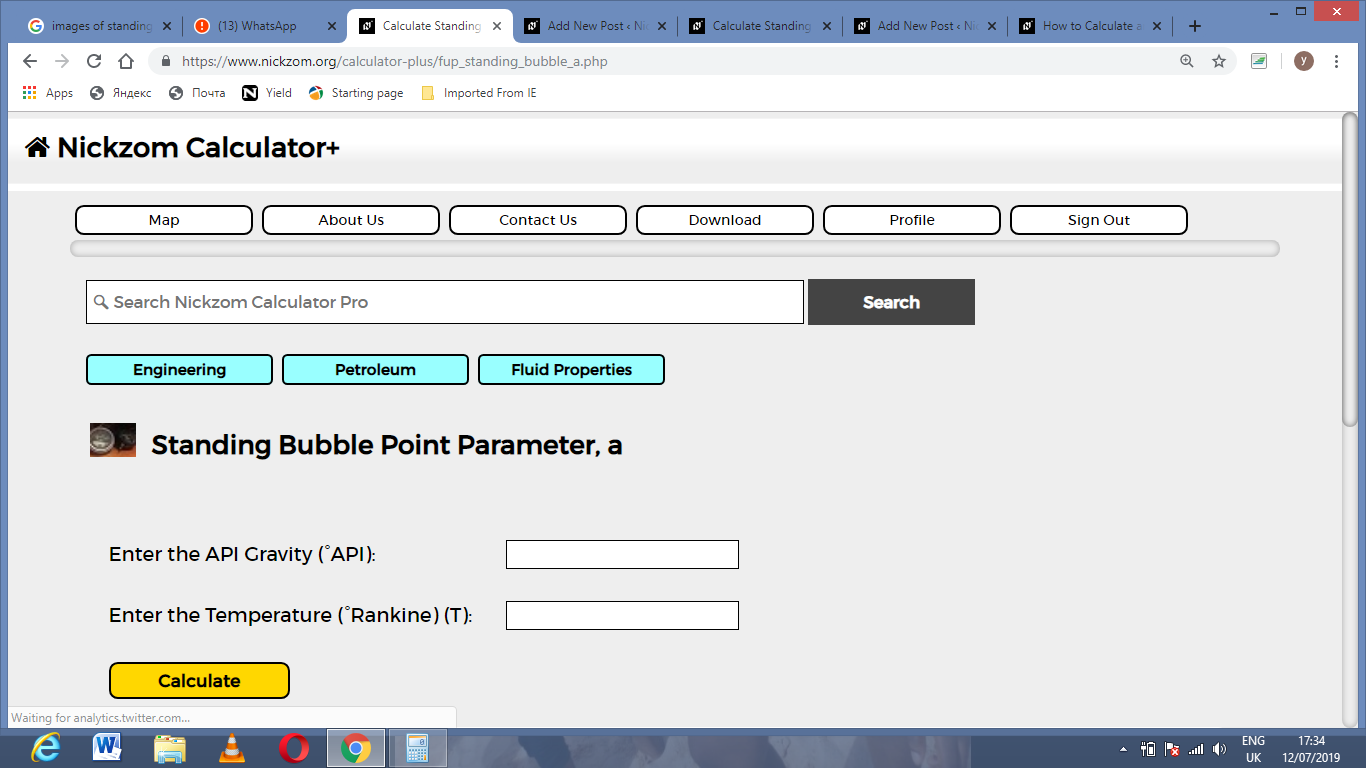Now, enter the values appropriately and accordingly for the parameters as required by the API Gravity (°API) is 32 and Temperature (°Rankine) (T). is 146.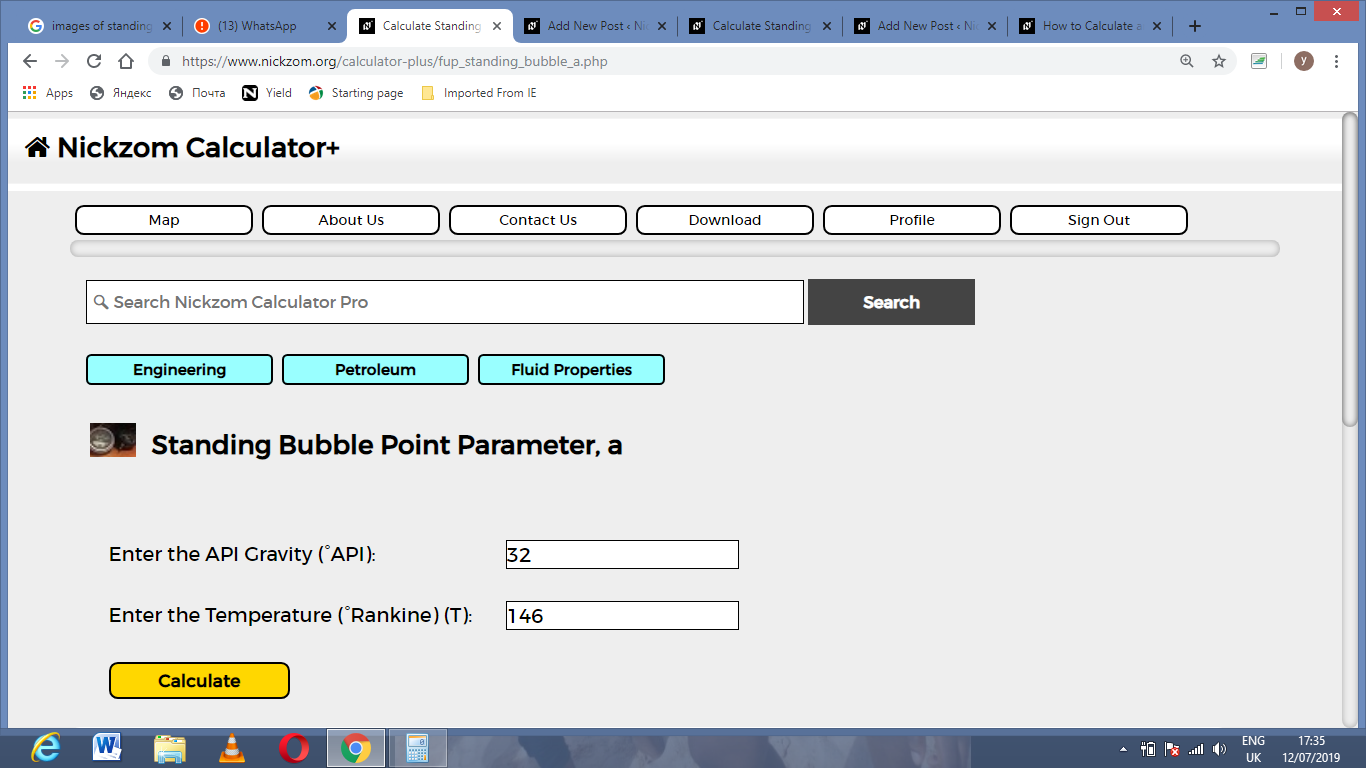Finally, Click on Calculate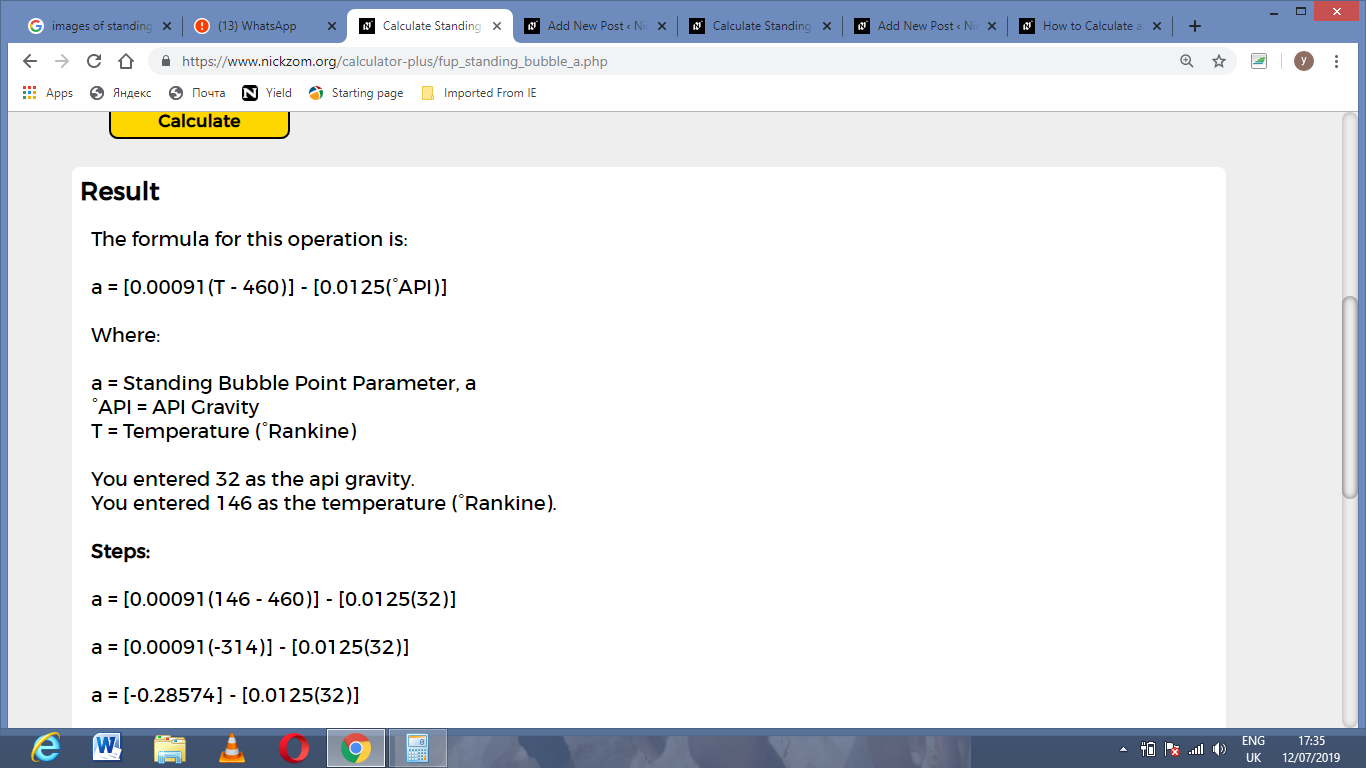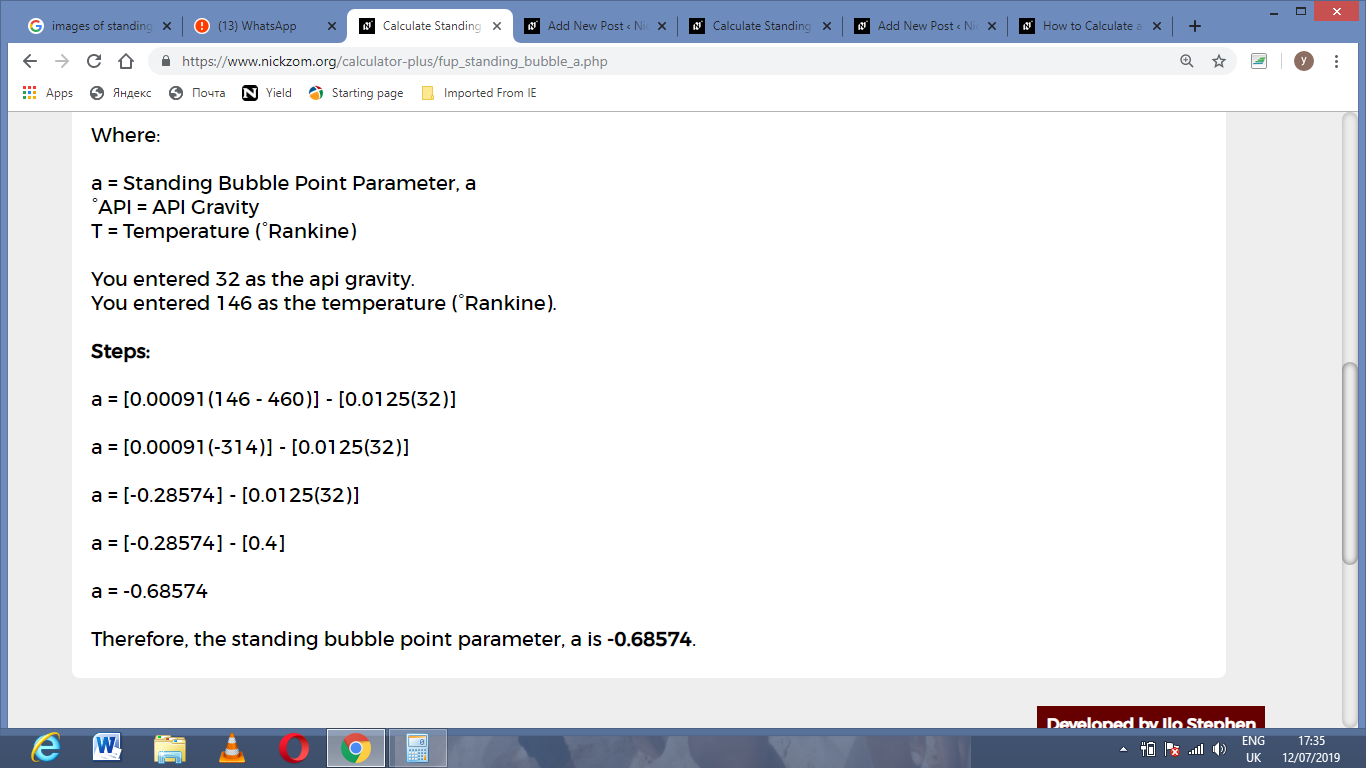As you can see from the screenshot above, Nickzom Calculator– The Calculator Encyclopedia solves for the standing bubble point parameter and presents the formula, workings and steps too.